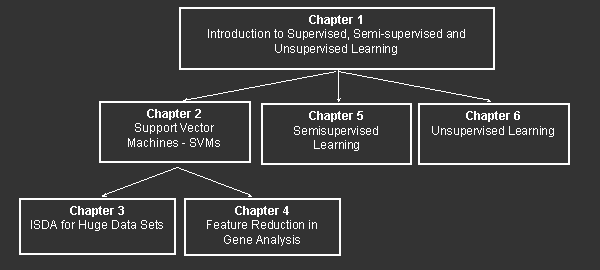# Kernel Based Algorithms for Mining Huge Data Sets

 Chapter SurveyThis book is divided into 6 chapters and the structure of the book is shown in the figure above.      The basic motivations and presentation of three different approaches in solving three unlike learning from data tasks are given in Chap. 1. It is a kind of both the background and the stage for a book to evolve. Chapter 2 introduces the constructive part of the SVMs without going into all the theoretical foundations of statistical learning theory which can be found in many other books. This may be particularly appreciated by and useful for the applications oriented readers who do not need to know all the theory back to its roots and motives. The basic quadratic programming (QP) based learning algorithms for both classification and regression problems are presented here. The ideas are introduced in a gentle way starting with the learning algorithm for classifying linearly separable data sets, through the classification tasks having overlapped classes but still a linear separation boundary, beyond the linearity assumptions to the nonlinear separation boundary, and finally to the linear and nonlinear regression problems. The appropriate examples follow each model derived, just enabling in this way an easier grasping of concepts introduced. The material provided here will be used and further developed in two specific directions in Chaps. 3 and 4. Chapter 3 resolves the crucial problem of the QP based learning coming from the fact that the learning stage of SVMs scales with the number of training data pairs. Thus, when having more than few thousands data pairs, the size of the original Hessian matrix appearing in the cost function of the QP problem setting goes beyond the capacities of contemporary computers. The fact that memory chips are increasing is not helping due to the much faster increase in the size of data files produced. Thus, there is a need for developing an iterative learning algorithm that does not require a calculation of the complete Hessian matrix. The Iterative Single Data Algorithm (ISDA) that in each iteration step needs a single data point only is introduced here. Its performance seems to be superior to other known iterative approaches. Chapter 4 shows how SVMs can be used as a feature reduction tools by coupling with the idea of recursive feature elimination. The Recursive Feature Elimination with Support Vector Machines (RFE-SVMs) developed in Guyon et all. (2002) is the first approach that utilizes the idea of margin as a measure of relevancy for feature selection. In this chapter, an improved RFE-SVM is also proposed and it is applied to the challenging problem of DNA microarray analysis. DNA microarray is a powerful tool which allows biologists to measure thousands of genes’ expression in a single experiment. This technology opens up the possibility of finding out the causal relationship between genes and certain phenomenon in the body, e.g. which set of genes is responsible for certain disease or illness. However, the high cost of the technology and the limited number of samples available make the learning from DNA microarray data a very difficult task. This is due to the fact that the training data set normally consists of a few dozens of samples, but the number of genes (i.e., the dimensionality of the problem) can be as high as several thousands. The results of applying the improved RFE-SVM to two DNA microarray data sets show that the performance of RFE-SVM seems to be superior to other knownapproaches such as the nearest shrunken centroid developed in Tibshirani et. all. (2002). back to top Chapter 5 presents two very promising semi-supervised learning techniques, namely, GRFM and CM. Both methods are based on the theory of graphical models and they explore the manifold structure of the data set which leads to their global convergence. An in depth analysis of both approaches when facing with unbalanced labeled suggests that the performance of both approaches can deteriorate very significantly when labeled data is unbalanced (i.e., when the number of labeled data in each class is different). As a result, a novel normalization step is introduced to both algorithms improving the performance of the algorithms very significantly when faced with an unbalance in labeled data. This chapter also presents the comparisons of CM and GRFM with the various variants of transductive SVMs (TSVMs) and the results suggest that the graph-based approaches seem to have better performance in multi-class problems. Chapter 6 introduces two basic methodologies for learning from unlabeled data within the unsupervised learning approach: the Principal Component Analysis (PCA) and the Independent Component Analysis (ICA). Unsupervised learning is related to the principle of redundancy reduction which is implemented in mathematical form through minimization of the statistical dependence between observed data pairs. It is demonstrated that PCA, which decorrelates data pairs, is optimal for Gaussian sources and suboptimal for non-Gaussian ones. It is also pointed to the necessity of using ICA for non-Gaussian sources as well as that there is no reason for using it in the case of Gaussian ones. PCA algorithm known as whitening or sphering transform is derived. Batch and adaptive ICA algorithms are derived through the minimization of the mutual information which is an exact measure of statistical (in)dependence between data pairs. Both PCA and ICA derived unsupervised learning algorithms are implemented in MATLAB code, which illustrates their use on computer generated examples. back to top